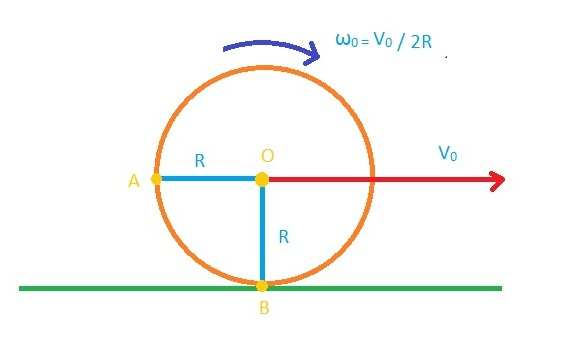# Rolling BallA spherical ball of mass M and radius R $slips$ on a horizontal plane. At some instant, it has translational velocity $v_{0}$ and rotational velocity about the centre O, $\frac {v_{0}}{2R}$. Then, the rotational velocity of the ball after it starts rolling perfectly is given by-

×